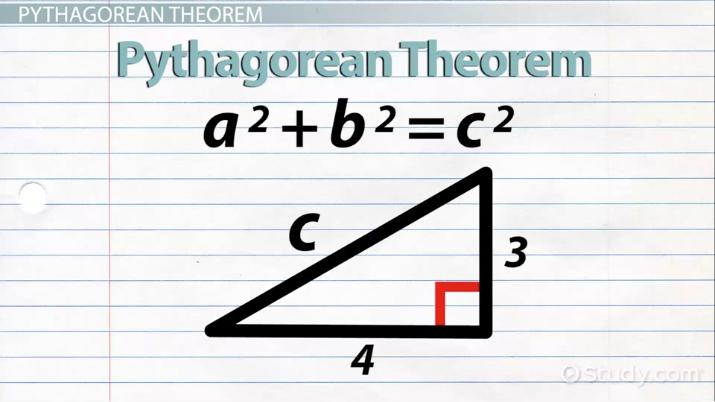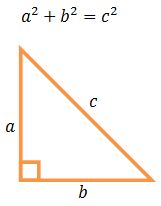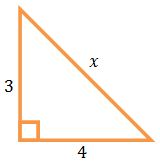# What is a Right Angle? - Definition & Formula

Lesson Transcript
Instructor: Yuanxin (Amy) Yang Alcocer

Amy has a master's degree in secondary education and has taught math at a public charter high school.

Right angles are all angles that are exactly 90 degrees and the most obvious in geometry is the triangle. Learn the definition of a right angle and explore the and notation and formula for right angles using the Pythagorean theorem, which relates all sides of a triangle to one another. Updated: 10/03/2021

## Definition of a Right Angle

A right angle is an angle that measures exactly 90 degrees. It is exactly a quarter of a circle. When you cut a pie into four equal pieces, the tip of each slice will form a right angle.Notice how the tips of each slice make a corner that is a right angle.An error occurred trying to load this video.

Try refreshing the page, or contact customer support.

Coming up next: What is a Straight Angle? - Definition & Example

### You're on a roll. Keep up the good work!

Replay
Your next lesson will play in 10 seconds
• 0:05 Definition of a Right Angle
• 0:22 Notation
• 1:15 Pythagorean Theorem
• 2:53 Lesson Summary
Save Save

Want to watch this again later?

Timeline
Autoplay
Autoplay
Speed Speed

## Notation

Right angles have a special notation all their own. It is a symbol that makes it look like a little square is in the angle. It looks like this:The little square box tells you that this angle is a right angle.

You will see right angles in many places. You will see them in squares and rectangles where each corner is a right angle. One of the most useful places you will see them is in triangles. When a triangle has a right angle, it becomes a special type of triangle called a right triangle. Right triangles always have exactly one angle that is 90 degrees. They look like this:Right triangles provide the basis for many mathematical things, such as finding the distance up a slope if you only know the height of the hill and determining how wide the hill is. Without right triangles, we wouldn't have trigonometry. The trigonometric functions of sine, cosine, and tangent all are based on the right triangle.

## Pythagorean Theorem

One of the most important formulas you will ever come across related to right triangles is the Pythagorean theorem. The Pythagorean theorem is the formula that relates all three sides of a right triangle to each other. It allows you to find one side given the other two.The side c is always the hypotenuse or the side opposite the right angle. The hypotenuse is always the longest side of a right triangle. The Pythagorean theorem tells you that the hypotenuse squared is the same length as the sum of the squares of the other two sides.

Let's see how we can use the Pythagorean theorem to help us solve problems. Let's say we had this triangle, and we wanted to find the missing side.To unlock this lesson you must be a Study.com Member.

### Register to view this lesson

Are you a student or a teacher?